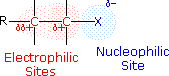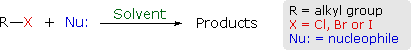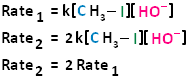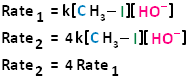Skip to main content

# 11.3: The SN2 Reaction

Objectives

After completing this section, you should be able to

1. write an expression relating reaction rate to the concentration of reagents for a second-order reaction.
2. determine the order of a chemical reaction from experimentally obtained rate data.
3. describe the essential features of the SN2 mechanism, and draw a generalized transition state for such a reaction.

Key Terms

Make certain that you can define, and use in context, the key terms below.

• bimolecular
• kinetics
• rate coefficient
• rate equation
• reaction rate
• second-order reaction
• SN2

Study Notes

Most of the key terms introduced in this section should already be familiar to you from your previous general chemistry course.

Reaction rate refers to the change in concentration of a reactant or product per unit of time. Using strict SI units, reaction rates are expressed in mol · L−1 · s−1, but in some textbooks you will find this value written as M/s. In general, the reaction rate of a given reaction changes with time, as it is dependent on the concentration of one or more of the reactants.

An equation which shows the relationship between the reaction rate and the concentrations of the reactants is known as the rate equation. All rate equations contain a proportionality constant, usually given the symbol k, which is known as the rate coefficient. Some textbooks refer to this value as the “rate constant,” but this name is a little misleading as it is not a true constant. The rate coefficient of a given reaction depends on such factors as temperature and the nature of the solvent.

SN2 is short for “bimolecular nucleophilic substitution.” You will encounter abbreviations for other types of reactions later in this chapter.

If you are unclear on the point about the inversion of configuration during an SN2 reaction, construct a molecular model of a chiral alkyl halide, the transition state formed when this substance reacts with a nucleophile in an SN2 process, and the product obtained from this reaction.

In many ways, the proton transfer process in a Brønsted-Lowry acid-base reaction can be thought of as simply a special kind of nucleophilic substitution reaction, one in which the electrophile is a hydrogen rather than a carbon.In both reaction types, we are looking at very similar players: an electron-rich species (the nucleophile/base) attacks an electron-poor species (the electrophile/proton), driving off the leaving group/conjugate base.

In the next few sections, we are going to be discussing some general aspects of nucleophilic substitution reactions, and in doing so it will simplify things greatly if we can use some abbreviations and generalizations before we dive into real examples.

Instead of showing a specific nucleophile like hydroxide, we will simply refer to the nucleophilic reactant as 'Nu'. In a similar fashion, we will call the leaving group 'X'. We will see as we study actual reactions that leaving groups are sometimes negatively charged, sometimes neutral, and sometimes positively charged. We will also see some examples of nucleophiles that are negatively charged and some that are neutral. Therefore, in this general picture we will not include a charge designation on the 'X' or 'Nu' species. In the same way, we will see later that nucleophiles and leaving groups are sometimes protonated and sometimes not, so for now, for the sake of simplicity, we will not include protons on 'Nu' or 'X'. We will generalize the three other groups bonded on the electrophilic central carbon as R1, R2, and R3: these symbols could represent hydrogens as well as alkyl groups. Finally, in order to keep figures from becoming too crowded, we will use in most cases the line structure convention in which the central, electrophilic carbon is not drawn out as a 'C'.

Here, then, is the generalized picture of a concerted (single-step) nucleophilic substitution reaction:The functional group of alkyl halides is a carbon-halogen bond, the common halogens being fluorine, chlorine, bromine and iodine. With the exception of iodine, these halogens have electronegativities significantly greater than carbon. Consequently, this functional group is polarized so that the carbon is electrophilic and the halogen is nucleophilic..Two characteristics other than electronegativity also have an important influence on the chemical behavior of these compounds.

1) The first of these is covalent bond strength. The strongest of the carbon-halogen covalent bonds is that to fluorine. Remarkably, this is the strongest common single bond to carbon, being roughly 30 kcal/mole stronger than a carbon-carbon bond and about 15 kcal/mole stronger than a carbon-hydrogen bond. Because of this, alkyl fluorides and fluorocarbons in general are chemically and thermodynamically quite stable, and do not share any of the reactivity patterns shown by the other alkyl halides. The carbon-chlorine covalent bond is slightly weaker than a carbon-carbon bond, and the bonds to the other halogens are weaker still, the bond to iodine being about 33% weaker.

2) The second factor to be considered is the relative stability of the corresponding halide anions, which is likely the form of the leaving group when these electronegative atoms are replaced. This stability may be estimated from the relative acidities of the H-X acids, assuming that the strongest acid releases the most stable conjugate base (halide anion). With the exception of HF (pKa = 3.2), all the hydrohalic acids are very strong, small differences being in the direction HCl < HBr < HI.

In order to understand why some combinations of alkyl halides and nucleophiles give a substitution reaction, whereas other combinations give elimination, and still others give no observable reaction, we must investigate systematically the way in which changes in reaction variables perturb the course of the reaction. The following general equation summarizes the factors that will be important in such an investigation.One conclusion, relating the structure of the R-group to possible products, should be immediately obvious. If R- has no beta-hydrogens an elimination reaction is not possible, unless a structural rearrangement occurs first. The first four halides shown on the left below do not give elimination reactions on treatment with base, because they have no β-hydrogens. The two halides on the right do not normally undergo such reactions because the potential elimination products have highly strained double or triple bonds.

It is also worth noting that sp2 hybridized C–X compounds, such as the three on the right, do not normally undergo nucleophilic substitution reactions, unless other functional groups perturb the double bond(s).Using the general reaction shown above as our reference, we can identify the following variables and observables.

Variables R change α-carbon from 1º to 2º to 3º if the α-carbon is a chiral center, set as (R) or (S) X change from Cl to Br to I (F is relatively unreactive) Nu: change from anion to neutral; change basicity; change polarizability Solvent polar vs. non-polar; protic vs. non-protic Products substitution, elimination, no reaction. Stereospecificity if the α-carbon is a chiral center what happens to its configuration? Reaction Rate measure as a function of reactant concentration.

When several reaction variables may be changed, it is important to isolate the effects of each during the course of study. In other words: only one variable should be changed at a time, the others being held as constant as possible. For example, we can examine the effect of changing the halogen substituent from Cl to Br to I, using ethyl as a common R–group, cyanide anion as a common nucleophile, and ethanol as a common solvent. We would find a common substitution product, C2H5–CN, in all cases, but the speed or rate of the reaction would increase in the order: Cl < Br < I. This reactivity order reflects both the strength of the C–X bond, and the stability of X(–) as a leaving group, and leads to the general conclusion that alkyl iodides are the most reactive members of this functional class.

### The SN2 mechanism

There are two mechanistic models for how an alkyl halide can undergo nucleophilic substitution. In the first picture, the reaction takes place in a single step, and bond-forming and bond-breaking occur simultaneously. (In all figures in this section, 'X' indicates a halogen substituent).This is called an 'SN2' mechanism. In the term SN2, S stands for 'substitution', the subscript N stands for 'nucleophilic', and the number 2 refers to the fact that this is a bimolecular reaction: the overall rate depends on a step in which two separate molecules (the nucleophile and the electrophile) collide. A potential energy diagram for this reaction shows the transition state (TS) as the highest point on the pathway from reactants to products.If you look carefully at the progress of the SN2 reaction, you will realize something very important about the outcome. The nucleophile, being an electron-rich species, must attack the electrophilic carbon from the back side relative to the location of the leaving group. Approach from the front side simply doesn't work: the leaving group - which is also an electron-rich group - blocks the way.The result of this backside attack is that the stereochemical configuration at the central carbon inverts as the reaction proceeds. In a sense, the molecule is turned inside out. At the transition state, the electrophilic carbon and the three 'R' substituents all lie on the same plane.What this means is that SN2 reactions whether enzyme catalyzed or not, are inherently stereoselective: when the substitution takes place at a stereocenter, we can confidently predict the stereochemical configuration of the product. Below is an animation illustrating the principles we have just learned, showing the SN2 reaction between hydroxide ion and methyl iodide. Notice how backside attack by the hydroxide nucleophile results in inversion at the tetrahedral carbon electrophile.Exercise

Predict the structure of the product in this SN2 reaction. Be sure to specify stereochemistry.We will be contrasting about two types of nucleophilic substitution reactions. One type is referred to as unimolecular nucleophilic substitution (SN1), whereby the rate determining step is unimolecular and bimolecular nucleophilic substitution (SN2), whereby the rate determining step is bimolecular. We will begin our discussion with SN2 reactions, and discuss SN1 reactions elsewhere.

## Bimolecular Nucleophilic Substitution Reactions and Kinetics

In the term SN2, (as previously stated) the number two stands for bimolecular, meaning there are two molecules involved in the rate determining step. The rate of bimolecular nucleophilic substitution reactions depends on the concentration of both the haloalkane and the nucleophile. To understand how the rate depends on the concentrations of both the haloalkane and the nucleophile, let us look at the following example. The hydroxide ion is the nucleophile and methyl iodide is the haloalkane.If we were to double the concentration of either the haloalkane or the nucleophile, we can see that the rate of the reaction would proceed twice as fast as the initial rate.If we were to double the concentration of both the haloalkane and the nucleophile, we can see that the rate of the reaction would proceed four times as fast as the initial rate.The bimolecular nucleophilic substitution reaction follows second-order kinetics; that is, the rate of the reaction depends on the concentration of two first-order reactants. In the case of bimolecular nucleophilic substitution, these two reactants are the haloalkane and the nucleophile. For further clarification on reaction kinetics, the following links may facilitate your understanding of rate laws, rate constants, and second-order kinetics:

## Frontside vs. Backside Attacks

A bimolecular nucleophilic substitution (SN2) reaction is a type of nucleophilic substitution whereby a lone pair of electrons on a nucleophile attacks an electron deficient electrophilic center and bonds to it, resulting in the expulsion of a leaving group. It is possible for the nucleophile to attack the electrophilic center in two ways.

• Frontside Attack: In a frontside attack, the nucleophile attacks the electrophilic center on the same side as the leaving group. When a frontside attack occurs, the stereochemistry of the product remains the same; that is, we have retention of configuration.
• Backside Attack: In a backside attack, the nucleophile attacks the electrophilic center on the side that is opposite to the leaving group. When a backside attack occurs, the stereochemistry of the product does not stay the same. There is inversion of configuration.

The following diagram illustrates these two types of nucleophilic attacks, where the frontside attack results in retention of configuration; that is, the product has the same configuration as the substrate. The backside attack results in inversion of configuration, where the product's configuration is opposite that of the substrate.Experimental Observation: All SN2 Reactions Proceed With Backside Attacks

Experimental observation shows that all SN2 reactions proceed with inversion of configuration; that is, the nucleophile will always attack from the backside in all SN2 reactions. To think about why this might be true, remember that the nucleophile has a lone pair of electrons to be shared with the electrophilic center, and the leaving group is going to take a lone pair of electrons with it upon leaving. Because like charges repel each other, the nucleophile will always proceed by a backside displacement mechanism.

## SN2 Reactions Are Stereospecific

The SN2 reaction is stereospecific. A stereospecific reaction is one in which different stereoisomers react to give different stereoisomers of the product. For example, if the substrate is an R enantiomer, a frontside nucleophilic attack results in retention of configuration, and the formation of the R enantiomer. A backside nucleophilic attack results in inversion of configuration, and the formation of the S enantiomer.Conversely, if the substrate is an S enantiomer, a frontside nucleophilic attack results in retention of configuration, and the formation of the S enantiomer. A backside nucleophilic attack results in inversion of configuration, and the formation of the R enantiomer.In conclusion, SN2 reactions that begin with the R enantiomer as the substrate will form the S enantiomer as the product. Those that begin with the S enantiomer as the substrate will form the R enantiomer as the product. This concept also applies to substrates that are cis and substrates that are trans. If the cis configuration is the substrate, the resulting product will be trans. Conversely, if the trans configuration is the substrate, the resulting product will be cis.

## Exercises

1. In an experiment to investigate the kinetics of the reaction

$\ce{\sf{CH3Cl + OH^{−} -> CH3OH + Cl^{−}}}$

the following results were obtained:

initial concentration of chloromethane = 0.01 mol · L−1
initial concentration of hydroxide ion = 0.01 mol · L−1
initial rate of reaction = 6 × 10−10 mol · L−1 · s−1

Assuming the reaction to be second order:

1. determine the value of the rate coefficient, k.
2. calculate the initial rate of the reaction if [CH3Cl]0 = 0.02 mol · L−1 and [OH]0 = 0.005 mol · L−1.

### Answer

If this reaction is an SN2 reaction as indicated in the question,

$\text{Rate}=k\left[{\text{CH}}_{3}\text{Cl}\right]\left[{\text{OH}}^{-}\right]$

1. Substituting the given values of the initial rate and concentrations

$6×{10}^{-10}\text{mol}\cdot {\text{L}}^{\text{-1}}\cdot {\text{s}}^{\text{-1}}=k\left(0.01\text{\hspace{0.17em}}\text{mol}\cdot {\text{L}}^{\text{-1}}\right)\left(0.01\text{\hspace{0.17em}}\text{mol}\cdot {\text{L}}^{\text{-1}}\right)$

or

$\begin{array}{rl}k& =\frac{6×{10}^{-10}\text{\hspace{0.17em}}}{}\end{array}$mol× L 1 s 1 (0.01mol L 1 )(0.01mol L 1 ) =6× 10 6 mol× L 1 s 1

2. Initial rate

$\begin{array}{rl}& =\left(6×{10}^{-6}\text{\hspace{0.17em}}\text{mol}\cdot {\text{L}}^{-1}\cdot {\text{s}}^{-1}\right)\left(0.02\text{\hspace{0.17em}}\text{mol}\cdot {\text{L}}^{-1}\right)\left(0.005\text{\hspace{0.17em}}\text{mol}\cdot {\text{L}}^{-1}\right)\\ & =\left(6×{10}^{-10}\text{\hspace{0.17em}}\text{mol}\cdot {\text{L}}^{-1}\cdot {\text{s}}^{-1}\right)\end{array}$